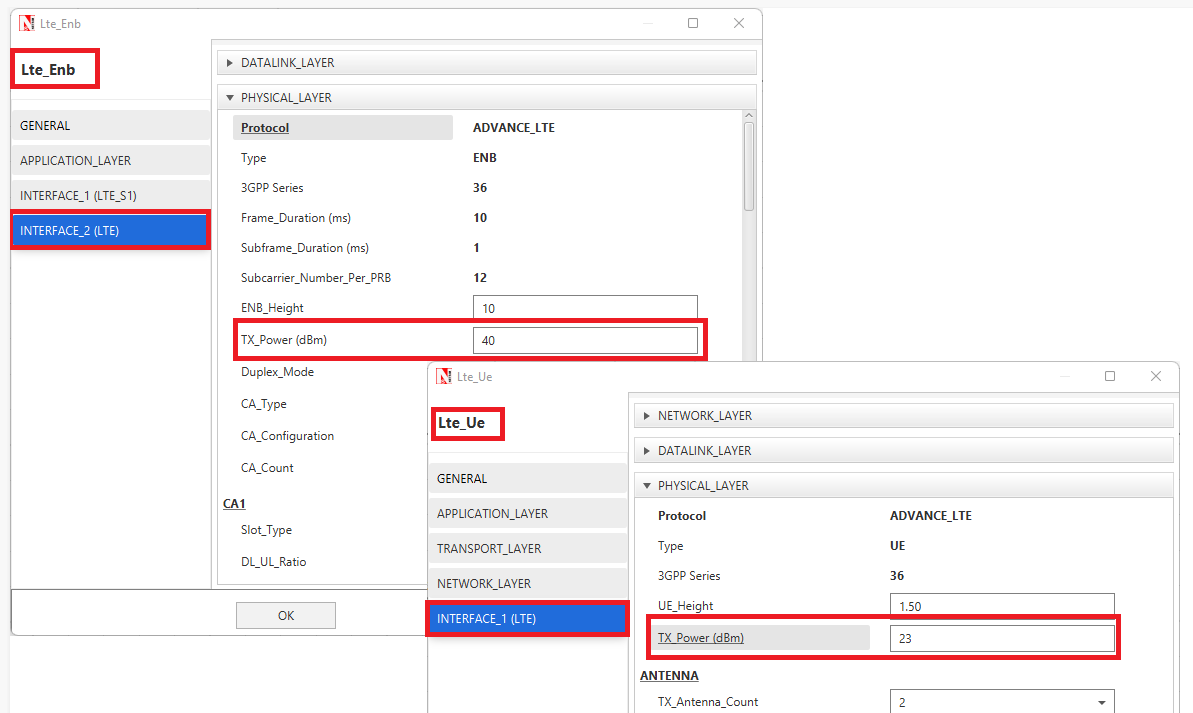Applicable Versions NetSim Standard NetSim Pro

The SNR is defined as the power of signal divided by the sum of the interference power and the power of background noise.

SINR(dBm) = log( Receivedpower(mW) / (Interference Noise(mW) + Thermal Noise(mW)))

The logarithm mentioned above is to the base 10

Transmit Power is an input parameter that can be set in the UI (eNB and UE properties).

The Transmit Power can be set by going to eNB/ UE properties-> Interface LTE-> Physical LayerApplicable Release v13

Thermal noise calculations is done in LTENR_Phy.c in the function LTENR_PHY_calculateThermalNoise()

Users can also refer to the functions LTENR_PHY_GetDownlinkSpectralEfficiency() and LTENR_PHY_GetUplinkSpectralEfficiency()  that is part of LTENR_Phy.c.

Calls to many of the functions mentioned above are made from here.

 Applicable Release v12

Received Power calculations are done in LTE_Phy.c in the function  fn_NetSim_LTE_CalculateRxPower()

Thermal noise calculations is done in LTE_Phy.c in the function fn_NetSim_LTE_CalculateSNR()

Users can also refer to the function fn_NetSim_LTE_CalculateReceivedPower() that is part of LTE_Phy.c.

Calls to many of the functions mentioned above are made from here.